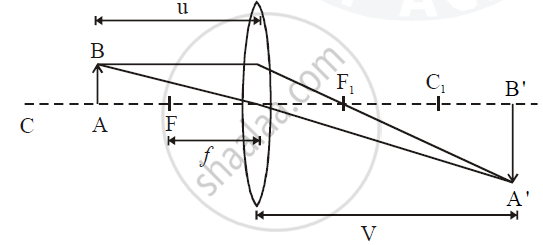Share

# Solution - An Object is Placed at a Distance of 12 Cm from a Convex Lens of Focal Length 8 Cm. Find : the Position of the Image and Nature of the Image - Refraction of Light - Image Formation in Lenses Using Ray Diagrams - Convex Lens

#### Question

An object is placed at a distance of 12 cm from a convex lens of focal length 8 cm. Find :

1) the position of the image

2) nature of the image

#### Solution

1)Given data:

u = -12 cm, f = +8 cm, v = ?

Lens formula

1/v - 1/u = 1/f

1/v - 1/(-12) = 1/8

1/v = 1/8 - 1/12

v = 24 cm [Position of Image]

2) Nature if image : Real, inverted and magnified.

Is there an error in this question or solution?
Solution An Object is Placed at a Distance of 12 Cm from a Convex Lens of Focal Length 8 Cm. Find : the Position of the Image and Nature of the Image Concept: Refraction of Light - Image Formation in Lenses Using Ray Diagrams - Convex Lens.
S